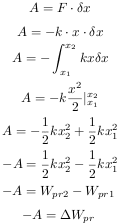# Deriving potential energy

71GA
Hello, i am trying to derive flexible energy, but i get a negative sign in front of a Work (A). Where did i miss?
F is force of a spring. I don't know if i used Hooke's law correctly...Thank you for looking.

Last edited:

71GA
Nomrally, potential energy is the negative of the work performed by some force in moving some object from one point to another.

http://en.wikipedia.org/wiki/Potential_energy

I am sorry i miss write... it is about flexible energy :)

nasu
I think you mean "elastic energy". And the elastic energy is a form of potential energy.
What you calculate is the work done by the elastic force between the points 1 and 2. This is equal to -(U2-U1).